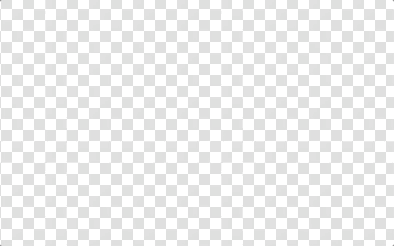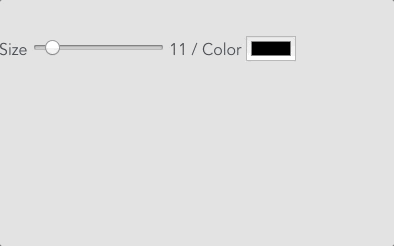# How to Draw Without Antialiasing on HTML5 Canvasdraw by native api (this isn’t what I want)draw on Pixilart (this isn’t what I want too because it shaped square)draw by stamp (it’s not too bad)
`this.context.drawImage(stampImage, Math.round(x), Math.round(y))// float number is one of the several causes of antialiasing`
`function distanceBetween (point1, point2) {  return Math.sqrt(Math.pow(point2.x - point1.x, 2) + Math.pow(point2.y - point1.y, 2))}function angleBetween (point1, point2) {  return Math.atan2(point2.x - point1.x, point2.y - point1.y)}const dist = distanceBetween(beginPosition, destPosition)const angle = angleBetween(beginPosition, destPosition)for (let i = 0; i < dist; i += 5) {  const x = beginPosition.x + (Math.sin(angle) * i) - halfSizeOfBrush  const y = beginPosition.y + (Math.cos(angle) * i) - halfSizeOfBrush  this.context.drawImage(stampImage, Math.round(x), Math.round(y))}`draw by stamp smoothly (this is what I wanted!)make stamps dynamically

Written by

## kozo yamagata

#### 東京でフリーランスのWebアプリケーションエンジニアをやっています。JavaScriptでリッチなUIを作るのが得意です。好きなものは音楽と料理とコーヒーです。I’m a web application engineer in tokyo.

Welcome to a place where words matter. On Medium, smart voices and original ideas take center stage - with no ads in sight. Watch
Follow all the topics you care about, and we’ll deliver the best stories for you to your homepage and inbox. Explore
Get unlimited access to the best stories on Medium — and support writers while you’re at it. Just \$5/month. Upgrade